A probabilistic roadmap (PRM) is a network graph of possible paths in a given map based on free and occupied spaces. The `mobileRobotPRM` object randomly generates nodes and creates connections between these nodes based on the PRM algorithm parameters. Nodes are connected based on the obstacle locations specified in `Map`, and on the specified `ConnectionDistance`. You can customize the number of nodes, `NumNodes`, to fit the complexity of the map and the desire to find the most efficient path. The PRM algorithm uses the network of connected nodes to find an obstacle-free path from a start to an end location. To plan a path through an environment effectively, tune the `NumNodes` and `ConnectionDistance` properties.

When creating or updating the `mobileRobotPRM` class, the node locations are randomly generated, which can affect your final path between multiple iterations. This selection of nodes occurs when you specify `Map` initially, change the parameters, or `update` is called. To get consistent results with the same node placement, use `rng` to save the state of the random number generation. See Tune the Connection Distance for an example using `rng`.

### Tune the Number of Nodes

Use the `NumNodes` property on the `mobileRobotPRM` object to tune the algorithm. `NumNodes` specifies the number of points, or nodes, placed on the map, which the algorithm uses to generate a roadmap. Using the `ConnectionDistance` property as a threshold for distance, the algorithm connects all points that do not have obstacles blocking the direct path between them.

Increasing the number of nodes can increase the efficiency of the path by giving more feasible paths. However, the increased complexity increases computation time. To get good coverage of the map, you might need a large number of nodes. Due to the random placement of nodes, some areas of the map may not have enough nodes to connect to the rest of the map. In this example, you create a large and small number of nodes in a roadmap.

Load a map file as a logical matrix, `simpleMaps`, and create an occupancy grid.

```load exampleMaps.mat map = binaryOccupancyMap(simpleMap,2);```

Create a simple roadmap with 50 nodes.

```prmSimple = mobileRobotPRM(map,50); show(prmSimple)```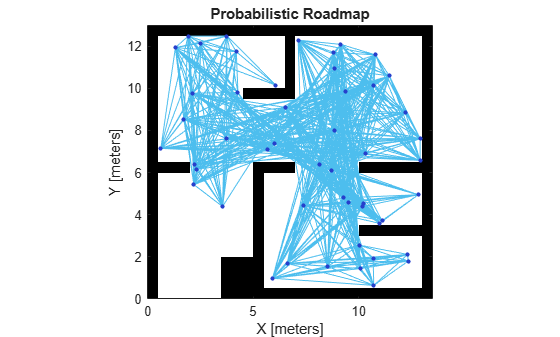Create a dense roadmap with 250 nodes.

```prmComplex = mobileRobotPRM(map,250); show(prmComplex)```The additional nodes increase the complexity but yield more options to improve the path. Given these two maps, you can calculate a path using the PRM algorithm and see the effects.

Calculate a simple path.

```startLocation = [2 1]; endLocation = [12 10]; path = findpath(prmSimple,startLocation,endLocation); show(prmSimple)```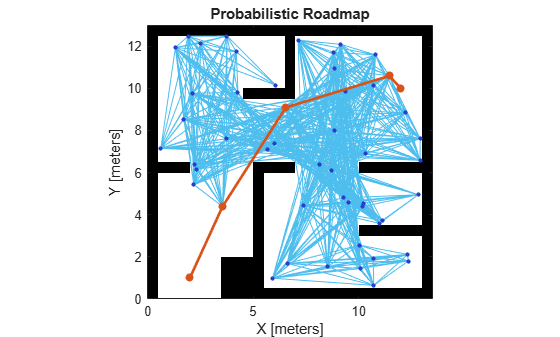Calculate a complex path.

```path = findpath(prmComplex, startLocation, endLocation); show(prmComplex)```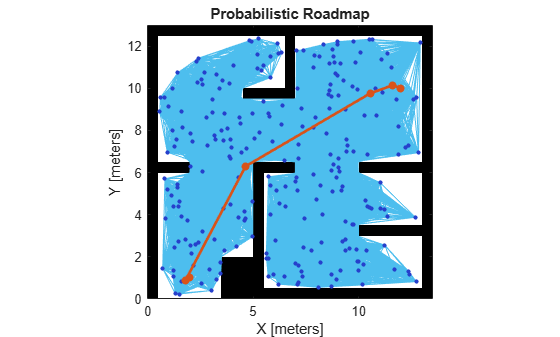Increasing the nodes allows for a more direct path, but adds more computation time to finding a feasible path. Because of the random placement of points, the path is not always more direct or efficient. Using a small number of nodes can make paths worse than depicted and even restrict the ability to find a complete path.

### Tune the Connection Distance

Use the `ConnectionDistance` property on the `PRM` object to tune the algorithm. `ConnectionDistance` is an upper threshold for points that are connected in the roadmap. Each node is connected to all nodes within this connection distance that do not have obstacles between them. By lowering the connection distance, you can limit the number of connections to reduce the computation time and simplify the map. However, a lowered distance limits the number of available paths from which to find a complete obstacle-free path. When working with simple maps, you can use a higher connection distance with a small number of nodes to increase efficiency. For complex maps with lots of obstacles, a higher number of nodes with a lowered connection distance increases the chance of finding a solution.

Load a map as a logical matrix, `simpleMap`, and create an occupancy grid.

```load exampleMaps.mat map = binaryOccupancyMap(simpleMap,2);```

Create a roadmap with 100 nodes and calculate the path. The default `ConnectionDistance` is set to inf. Save the random number generation settings using the rng function. The saved settings enable you to reproduce the same points and see the effect of changing `ConnectionDistance`.

```rngState = rng; prm = mobileRobotPRM(map,100); startLocation = [2 1]; endLocation = [12 10]; path = findpath(prm,startLocation,endLocation); show(prm)```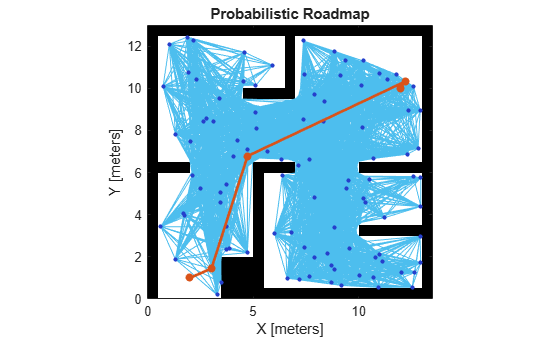Reload the random number generation settings to have PRM use the same nodes. Lower `ConnectionDistance` to 2 m. Show the calculated path.

```rng(rngState); prm.ConnectionDistance = 2; path = findpath(prm,startLocation,endLocation); show(prm)```### Create or Update PRM

When using the `mobileRobotPRM` object and modifying properties, with each new function call, the object triggers the roadmap points and connections to be recalculated. Because recalculating the map can be computationally intensive, you can reuse the same roadmap by calling `findpath` with different starting and ending locations.

Load the map, `simpleMap`, from a `.mat` file as a logical matrix and create an occupancy grid.

```load('exampleMaps.mat') map = binaryOccupancyMap(simpleMap,2);```

Create a roadmap. Your nodes and connections might look different due to the random placement of nodes.

```prm = mobileRobotPRM(map,100); show(prm)```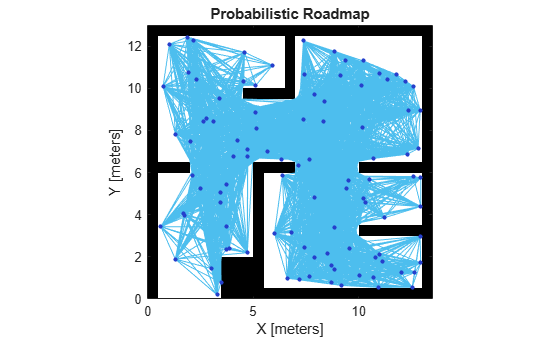Call `update` or change a parameter to update the nodes and connections.

```update(prm) show(prm)```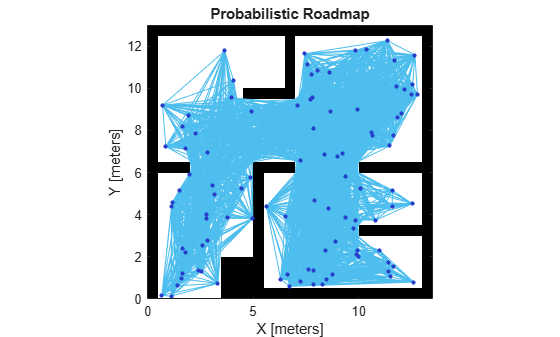The PRM algorithm recalculates the node placement and generates a new network of nodes.

 Kavraki, L.E., P. Svestka, J.-C. Latombe, and M.H. Overmars. "Probabilistic roadmaps for path planning in high-dimensional configuration spaces," IEEE Transactions on Robotics and Automation. Vol. 12, No. 4, Aug 1996 pp. 566—580.